# Image Formation by Lenses and the Eye

Image formation by a lens depends upon the wave property called refraction. Refraction may be defined as the bending of waves when they enter a medium where their speed is different. Since the speed of light is slower in a glass lens than in air, a light ray will be bent upon entering and upon exiting a lens in a way that depends upon the shape and curvature of the lens. In the case of a converging lens such as the double convex lens shown below, parallel rays will be brought together at a point.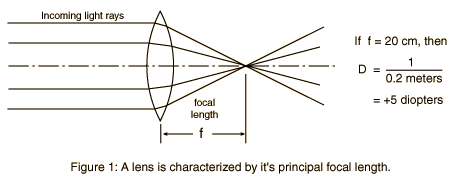The distance from the lens to this principal focus point is called the focal length of the lens and will be designated by the symbol f. A converging lens may be used to project an image of a lighted object. For example, the converging lens in a slide projector is used to project an image of a photographic slide on a screen, and the converging lens in the eye of the viewer in turn projects an image of the screen on the retina in the back of the eye.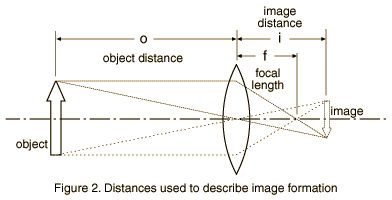There is a geometrical relationship between the focal length of a lens (f), the distance from the lens to the bright object (o) and the distance from the lens to the projected image (i). The relationship between the distances illustrated in Figure 2 can be expressed as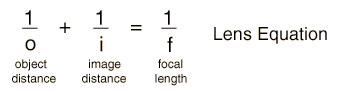This relationship will be used to determine the focal length of a glass lens, and will be used as the basis for a qualitative investigation of image formation by the eye with the use of a large eye model.

1. Position the lens and white screen on the optical bench and place them so that the distance from the lighted "object" to the lens can be measured on the bench scale. Adjust the screen to get a clear image.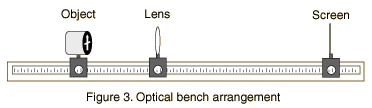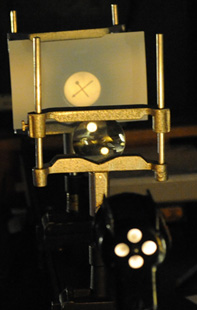Determine the object distance and image distance, o and i, and calculate the focal length from the lens relationship. Describe the appearance of the image, compared to the object (e.g, larger, smaller, erect, inverted). Adjust the object distance to a different value and repeat the process with a different set of measurements.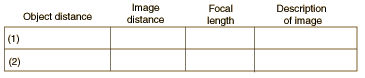2. What is the average of your focal length measurements, expressed in meters?

3. The lens strength in diopters is defined as S = 1/f(in meters). The unit is 1/m but this unit is commonly called a "diopter".

What is the strength of your lens in diopters?

Note: Converging lenses are listed as + and diverging lenses as - when diopter numbers are quoted. Prescriptions for eyeglasses are usually expressed in diopters.

I. Image formation by a lens; determination of focal length.

4. What is the diopter number of a lens of focal length 0.05 meters? What is the focal length of a +7 diopter lens?

5. Using the average value of your focal length measurements, calculate the image distance i if the object distance is 1.5 x your focal length (e.g, if your measured focal length were 10 cm, you would do the calculation for an object distance of 15 cm). For this arrangement, the image distance and linear magnification of the image are given by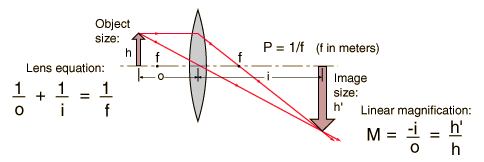Measure the height of your object and predict the size of the image which would be formed with the object placed as described above. Now make the actual measurements of image distance and image size and compare them with your predictions.

 Predictions Measured focal length f = _______ cm Object distance o = 1.5 x f = _______ cm Predicted image distance i = _______ cm Predicted magnification M = i/o = _______ cm Measurements Measured object size h = _______ cm Measured image distance i = _______ cm Measured image size = h' = _______ cm Predicted image size h' = _______ cm

II. Investigation of image formation by the eye.

The figure shows a sketch of the eye model. At the beginning of the experiment the retina should be in the middle, or "normal eye" position. The model should be filled with water to a height which covers the fixed lens of the eye model. This fixed lens of the eye model represents the cornea. In addition to the cornea, the human eye has another internal lens.

1. Place the object light about two meters away from the eye model so that light passes through the eye's lens and falls on the retina. Find a lens to place in the holder inside the water of the eye model which will give you a clear image on the retina. This internal lens in the human eye has a variable focal length and is changed in shape to allow you to change your eye's focus from a distant object to a close one. This change of focus is called "accommodation".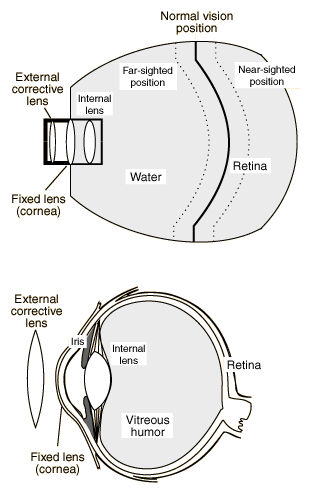Lens strength for sharp image at 2 meters: _________ 2. Move the object to a distance 35 cm from the front lens. To illustrate accommodation, and try to find a lens to replace the lens in the water which will again give you a clear image on the retina. Then make small adjustments in the object distance to get the sharpest possible image and record the object distance and lens strength needed to achieve it. Lens strength for sharp image at 35 cm : _________ If you moved it away from 35 cm, record the data: Distance for sharpest focus _____________ Lens strength ___________ Note: You should now have a clear image on the retina. The positions of the object and the eye model should be kept fixed for the next two steps of the experiment. 3. To simulate far-sightedness (hyperopia), move the retina of the eye model to the forward position. The light rays should be seen to converge toward a point behind the retina. Find a corrective lens for this defect. (Lenses for correction must be positioned in the slots outside the eye model.). Diopter number for best correction ___________

4. To simulate near-sightedness (myopia), move the retina to the rear. The light rays should be seen to converge toward a point in front of the retina. Find a corrective lens for this defect.

Corrective lens _______________

5. Remove the corrective lens from the front of the near-sighted eye model and replace it with the lens holder which has a metal plate with an aperture in it. Describe any changes in the image quality when this aperture is placed at the front of the eye model. Would a nearsighted person see clearer images in dim light or in bright light? Why do nearsighted persons squint?

6. The human eye is relaxed when it is focused on distant objects. From your experience in this lab, does the lens focal length approach a maximum or a minimum when your eye relaxes? Would this imply that the eye's lens is more rounded or less rounded when the eye muscle is relaxed?

## Equipment: Image Formation

• Optical bench
• Converging lens and lens holder
• Illuminated image and optical bench stand to hold it.
• White card for screen and stand to hold card
• Eye model including lens set
• Large illuminated object for eye model.
Index

Apparatus movie
HyperPhysics***** Physics 7120 ***** Physics 7120 Laboratory Go Back